International
Tables for
Crystallography
Volume C
Mathematical, physical and chemical tables
Edited by E. Prince

International Tables for Crystallography (2006). Vol. C, ch. 8.2, pp. 691-692

## Section 8.2.3. Entropy maximization

E. Princea and D. M. Collinsb

aNIST Center for Neutron Research, National Institute of Standards and Technology, Gaithersburg, MD 20899, USA, and bLaboratory for the Structure of Matter, Code 6030, Naval Research Laboratory, Washington, DC 20375-5341, USA

### 8.2.3. Entropy maximization

| top | pdf |

#### 8.2.3.1. Introduction

| top | pdf |

Entropy maximization, like least squares, is of interest primarily as a framework within which to find or adjust parameters of a model. Rationalization of the name entropy maximization' by analogy to thermodynamics is controversial, but there is formal proof (Shore & Johnson, 1980, Johnson & Shore, 1983) supporting entropy maximization as the unique method of inference that satisfies basic consistency requirements (Livesey & Skilling, 1985). The proof consists of discovering the consequences of four consistency axioms, which may be stated informally as follows:

 (1) the result of the inference should be unique; (2) the result of the inference should be invariant to any transformations of coordinate system; (3) it should not matter whether independent information is accounted for independently or jointly; (4) it should not matter whether independent subsystems are treated separately in conditional problems or collected and treated jointly.

The term entropy' is used in this chapter as a name only, the name for variation functions that include the form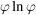, wheremay represent probability or, more generally, a positive proportion. Any positive measure, either observed or derived, of the relative apportionment of a characteristic quantity among observations can serve as the proportion.

The method of entropy maximization may be formulated as follows: given a set of n observations,, that are measurements of quantities that can be described by model functions,, where x is a vector of parameters, find the prior, positive proportions,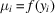, and the values of the parameters for which the positive proportions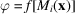make the sum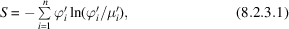where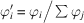and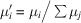, a maximum. S is called the Shannon–Jaynes entropy. For some applications (Collins, 1982), it is desirable to include in the variation function additional terms or restraints that give S the form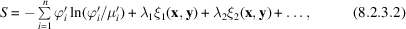where the λs are undetermined multipliers, but we shall discuss here only applications where λi = 0 for all i, and an unrestrained entropy is maximized. A necessary condition for S to be a maximum is for the gradient to vanish. Using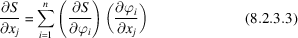and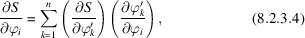straightforward algebraic manipulation gives equations of the form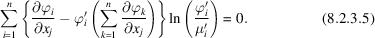It should be noted that, although the entropy function should, in principle, have a unique stationary point corresponding to the global maximum, there are occasional circumstances, particularly with restrained problems where the undetermined multipliers are not all zero, where it may be necessary to verify that a stationary solution actually maximizes entropy.

#### 8.2.3.2. Some examples

| top | pdf |

For an example of the application of the maximum-entropy method, consider (Collins, 1984) a collection of diffraction intensities in which various subsets have been measured under different conditions, such as on different films or with different crystals. All systematic corrections have been made, but it is necessary to put the different subsets onto a common scale. Assume that every subset has measurements in common with some other subset, and that no collection of subsets is isolated from the others. Let the measurement of intensity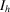in subset i be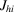, and let the scale factor that puts intensityon the scale of subset i be. Equation (8.2.3.1)becomes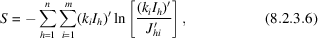where the term is zero ifdoes not appear in subset i. Becauseandare parameters of the model, equations (8.2.3.5)become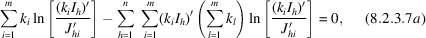and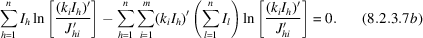These simplify to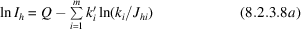and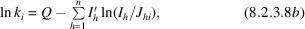where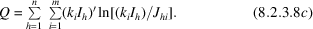Equations (8.2.3.8)may be solved iteratively, starting with the approximations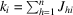and Q = 0.

The standard uncertainties of scale factors and intensities are not used in the solution of equations (8.2.3.8), and must be computed separately. They may be estimated on a fractional basis from the variances of estimated population means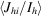for a scale factor and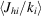for an intensity, respectively. The maximum-entropy scale factors and scaled intensities are relative, and either set may be multiplied by an arbitrary, positive constant without affecting the solution.

For another example, consider the maximum-entropy fit of a linear function to a set of independently distributed variables. Letrepresent an observation drawn from a population with mean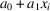and finite variance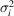; we wish to find the maximum-entropy estimate ofand. Assume that the mismatch between the observation and the model is normally distributed, so that its probability density is the positive proportion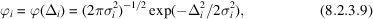where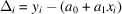. The prior proportion is given by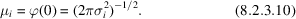Letting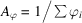, equations (8.2.3.5)become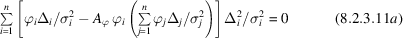and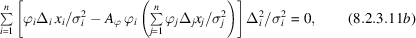which simplifies to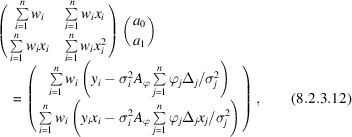wheremay be interpreted as a weight and is given by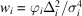. Equations (8.2.3.12)may be solved iteratively, starting with the approximations that the sums over j on the right-hand side are zero and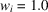for all i, that is, using the solutions to the corresponding, unweighted least-squares problem. Resettingafter each iteration by only half the indicated amount defeats a tendency towards oscillation. Approximate standard uncertainties for the parameters,and, may be computed by conventional means after setting to zero the sums over j on the right-hand side of equations (8.2.3.12). (See, however, a discussion of computing variance–covariance matrices in Section 8.1.2.) Note thatis small for both small and large values of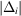. Thus, in contrast to the robust/resistant methods (Section 8.2.2), which de-emphasize only the large differences, this method down-weights both the small and the large differences and adjusts the parameters on the basis of the moderate-size mismatches between model and data. The procedure used in this two-dimensional, linear model can be extended to linear models, and linear approximations to nonlinear models, in any number of dimensions using methods discussed in Chapter 8.1.

The maximum-entropy method has been described (Jaynes, 1979) as being maximally noncommittal with respect to all other matters; it is as uniform (by the criterion of the Shannon information measure) as it can be without violating the given constraint[s]'. Least squares, because it gives minimum variance estimates of the parameters of a model, and therefore of all functions of the model including the predicted values of any additional data points, might be similarly described as maximally committal' with regard to the collection of more data. Least squares and maximum entropy can therefore be viewed as the extremes of a range of methods, classified according to the degree of a priori confidence in the correctness of the model, with the robust/resistant methods lying somewhere in between (although generally closer to least squares). Maximum-entropy methods can be used when it is desirable to avoid prejudice in favour of a model because of doubt as to the model's correctness.

### References

Collins, D. M. (1982). Electron density images from imperfect data by iterative entropy maximization. Nature (London), 298, 49–51.
Collins, D. M. (1984). Scaling by entropy maximization. Acta Cryst. A40, 705–708.
Jaynes, E. T. (1979). Where do we stand on maximum entropy? The maximum entropy formalism, edited by R. D. Liven & M. Tribus, pp. 44–49. Cambridge, MA: Massachusetts Institute of Technology.
Johnson, R. W. & Shore, J. E. (1983). Comments on and correction to 'Axiomatic derivation of the principle of maximum entropy and the principle of minimum cross-entropy'. IEEE Trans. Inf. Theory, IT-29, 942–943.
Livesey, A. K. & Skilling, J. (1985). Maximum entropy theory. Acta Cryst. A41, 113–122.
Shore, J. E. & Johnson, R. W. (1980). Axiomatic derivation of the principle of maximum entropy and the principle of minimum cross-entropy. IEEE Trans. Inf. Theory, IT-26, 26–37.